Tom Kelliher, CS 315

Apr. 5, 1999

### From Last Time

Segmented prefix problem.

### Coming Up

Multiplication and convolution.

# Previous Results

1. Add two k-bit numbers in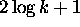bit steps.

2. Suppose we wish to add N k-bit numbers as quickly as possible.
1. What is the organization of the adder?

2. Do the adders become wider as we progress?

3. Running time:Are these really equivalent?

Can we do better?

1. Idea: Add three k-bit numbers in a single bit step, producing two k+1-bit numbers.

2. Implementation: Use a binary full adder for each bit position, producing a low order bit and a carry bit for each bit position.

3. The final sum is the sum of the low order bits and the shifted carry bits.

4. Example:5. Time required to reduce the sum of N k-bit numbers to the sum of two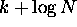-bit numbers: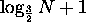bit steps.

Proof:

1. After the first step of CSA, how many numbers are we left with? (At most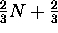. Why?)

2. After the second step?

3. After the jth step: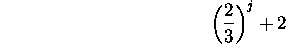4. After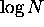steps, we're left with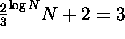numbers, so we need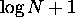steps to reduce to two-bit numbers.

6. Use a carry lookahead adder to reduce the two-number redundant representation to a single, non-redundant number.

7. Example: Draw the Wallace tree for N = 9.

Thomas P. Kelliher
Mon Apr 5 11:02:53 EDT 1999
Tom Kelliher